斯坦福大学机器学习笔记——降维（PCA算法）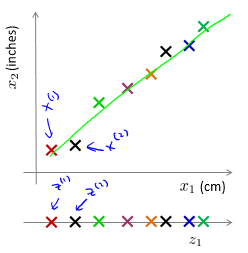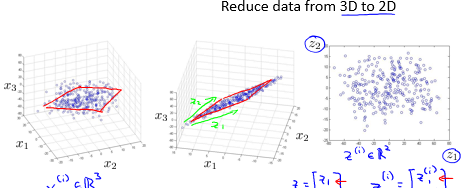1. 数据的压缩。从上面例子中可以看出，明显对数据进行了压缩。
2. 数据的可视化。对于我们来说我们最多能够可视化一个三维的画面，但是有些数据的维度大于三维，使得我们没办法可视化，但是使用降维的办法可以实现将原来的数据将至三维、二维甚至一维，从而有助于可视化显示。但是降维之后产生新特征的意义就必须有我们自己去发现了。

PCA是最常见的降维算法。它的思想为：找到一个由一系列方向向量组成的低维平面，当我们把所有的数据投射到该低维平面时，我们希望投射平均均方误差尽可能地小。投射误差是从特征向量向该低维平面做垂线的长度。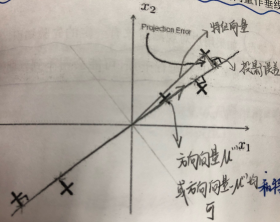PCA方法的问题描述：

PCA算法的过程如下：
1. 均值归一化（特征的缩放）。需要计算出所有特征的均值，然后令xj=xjuj$x_{j}=x_{j}-u_{j}$，同时如果特征是不同数量级上，我们还需要将其除以标准差。
2. 计算协方差矩阵Σ$\Sigma$:

Σ=1mi=1m(xi)(xi)T

3.计算协方差矩阵Σ$\Sigma$的特征值：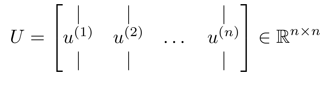4. 获取符合要求的新的特征向量。

zi=UTreduce×x(i)

f=1mi=1m||xixiapprox||2

g=1mi=1m||xi||2

1. 先令k=1，然后进行PCA，分别计算出Ureduce,z(1),z(2),...,z(m),x(1)approx,x(2)approx,...,x(m)approx$U_{reduce},z^{(1)},z^{(2)},...,z^{(m)},x_{approx}^{(1)},x_{approx}^{(2)},...,x_{approx}^{(m)}$检查是否fg0.01$\frac{f}{g}\leq 0.01$，如果满足则选择k=1，如果不满足，则令k=2，重复上述步骤，直到找到满足这个比例的k为止；
2. 还有一种更好的选择k值的方法：在我们求解协方差矩阵的特征值时，我们采用了[U,S,V]=svd(sigma)，其中S是一个n×n$n\times n$的矩阵，只有对角线上有值，而其它位置均为0，我们可以利用这个矩阵来计算该比例：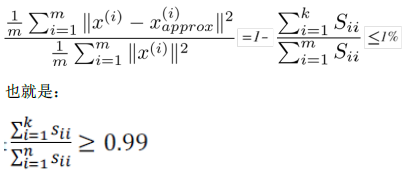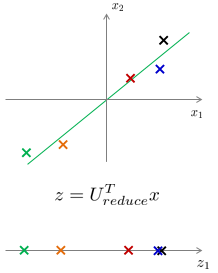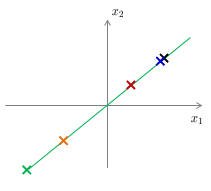1. 运用主成分分析将数据压缩至1000个特征
2. 然后对训练样本集运行学习算法（逻辑回归、svm、神经网络等）
3. 在预测时，采用之前学习而来的Ureduce$U_{reduce}$将输入的特征x转化成特征向量z，然后进行预测。

x(1),x(2),...,x(m)R10000PCAz(1),z(2),...,z(m)R1000

(z(1),y(1)),(z(2),y(2)),...,(z(m),y(m))algorithmhθ(z)=11+eθTz

xPCAzhθ(z)

1. PCA不是缓解过拟合的方法，对于缓解过拟合仍然采用正则化的思想。原因在于主成分分析只是近似地丢弃掉一些特征，它不会考虑任何与结果变量有关的信息(因为PCA是一种无监督的方法，不会使用标签信息)，因此可能会丢失非常重要的特征。对于正则化来说，会考虑结果变量(充分使用标签信息)，不会丢掉重要的特征。
2. 不要默认的将PCA一定是学习算法的某一部分，应该首先使用原始数据集用于学习算法，当用原始数据集学习算法运行的很慢或者内存和硬盘占用很高时，在考虑使用PCA。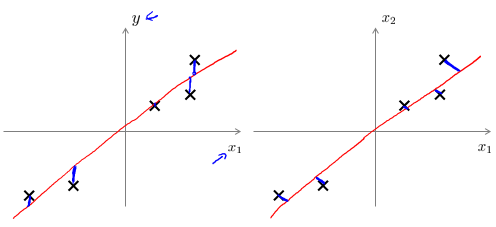PCA降维的优缺点：

1. 对数据进行降维处理。PCA根据协方差矩阵特征值的大小进行排序，根据需要取前面最重要的部分，将后面的维度省去，可以达到降维从而简化模型或是对数据进行压缩的效果，同时又最大程度的保持了原有数据的信息。
2. 完全无参数限制。完全不需要人为的设定参数或是根据任何经验模型对计算进行干预，最后结果只与数据相关，与用户独立